# Differential inequality

(diff) ← Older revision | Latest revision (diff) | Newer revision → (diff)

An inequality which interconnects the argument, the unknown function and its derivatives, e.g.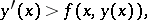(1)

whereis an unknown function of the argument. The principal problem in the theory of differential inequalities is to describe, starting from a known differential inequality and additional (initial or boundary) conditions, all its solutions.

Differential inequalities obtained from differential equations by replacing the equality sign by the inequality sign — which is equivalent to adding some non-specified function of definite sign to one of the sides of the equation — form a large class. A comparison of the solutions of such inequalities with the solutions of the corresponding differential equations is of interest. Thus, the following estimates  are valid for any solution of (1):(2)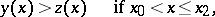whereon any intervalof existence of both solutions. This simple statement is extensively employed in estimating the solutions of differential equations (by passing to the respective differential inequality with a particular solution which is readily found), the domain of extendability of solutions, the difference between two solutions, in deriving conditions for the uniqueness of a solution, etc. A similar theorem  is also valid for a differential inequality (Chaplygin's inequality) of the type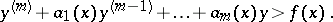Here, estimates of the type (2) for solutions satisfying identical initial conditions at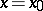are only certainly true on some interval determined by the coefficients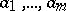. E.g., this is the intervalfor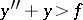.

For a system of differential inequalities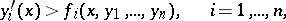it has been shown  that if each functionis non-decreasing with respect to the arguments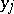(for all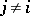), the estimate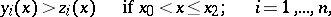resembling (2), is valid. The development of these considerations leads to the theory of differential inequalities in spaces with a cone.

A variant of differential inequalities is the requirement that the total derivative of a given function is of constant sign: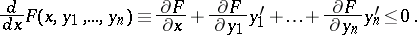This requirement is used in stability theory.

A representative of another class is the differential inequality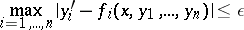(3)

(is given), which was first studied in the context of the general idea of an approximate description of a real problem by differential equations . Here the description of the integral funnel, i.e. the set of all points of all solutions which satisfy the given initial conditions, in particular, the behaviour of the funnel as, is of interest. A natural generalization of the differential inequality (3) is a differential equation in contingencies, specified by a field of cones, which generalizes the concept of a field of directions.

The theory of boundary value problems was also studied for differential inequalities. The inequality, whereis the Laplace operator, defines subharmonic functions; the differential inequality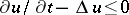defines subparabolic functions. Studies were also made of differential inequalities of a more general type (in both the above classes) with partial derivatives for differential operators of various types.

How to Cite This Entry:
Differential inequality. Encyclopedia of Mathematics. URL: http://encyclopediaofmath.org/index.php?title=Differential_inequality&oldid=17822
This article was adapted from an original article by A.D. Myshkis (originator), which appeared in Encyclopedia of Mathematics - ISBN 1402006098. See original article